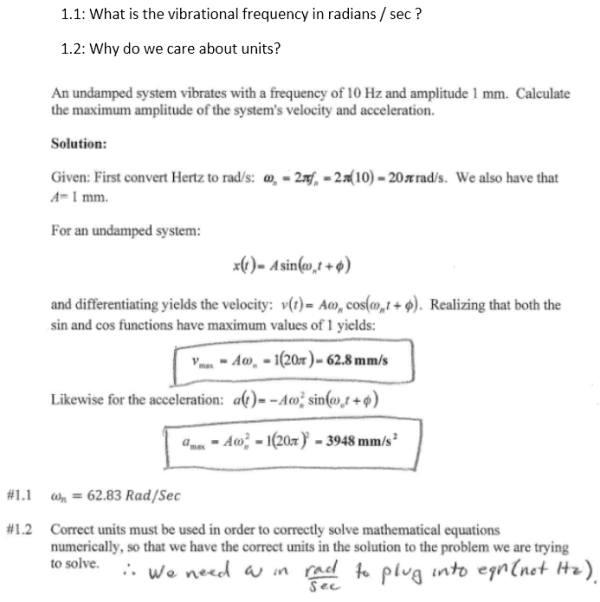An undamped system vibrates with a frequency of 10 Hz and amplitude 1 mm. Calculate the maximum amplitude of the system's velocity and acceleration. What is the vibrational frequency in radians/sec? Why do we care about units?An undamped system vibrates with a frequency of 10 Hz and amplitude 1 mm. Calculate the maximum amplitude of the system's velocity and acceleration. What is the vibrational frequency in radians/sec? Why do we care about units?

Vibrations Page 1 vibrations vibrations vibrations vibrations vibrations vibrations vibrations Vibrations Page 2